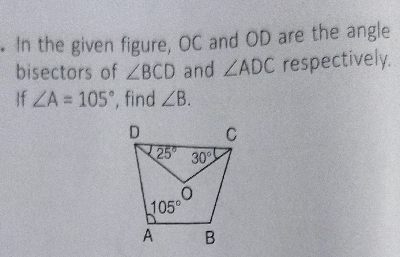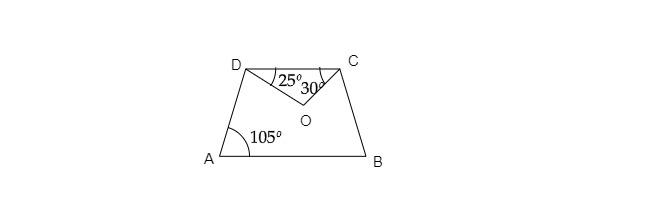"
">

# In the given figure, $O C$ and $O D$ are the angle bisectors of $\angle B C D$ and $\angle A D C$ respectively. If $\angle A=105^{\circ}$, find $\angle B$."

Given: In the given figure, $OC$ and $OD$ are the angle bisectors of $\angle BCD$ and $\angle ADC$ respectively and $\angle A=105^{\circ}$.

To do: To find $\angle B$.

Solution:$\because OD$ is the bisector of $ADC$

$\therefore OC$ is the bisector of $BCD$

$\Rightarrow \angle ADC=\angle D=\angle ODC\times 2$

$\Rightarrow \angle D=25^o\times 2=50^o$

Similarly: $\angle BCD=\angle C=OCD\times 2$

$\Rightarrow \angle C=30^o\times 2=60^o$

It is known that $A+B+C+D=360^o$    $( sum\ of\ angles\ of\ quadrilateral=360^o )$

$\Rightarrow 105^o+B+60^o+50^o=360^o$

$\Rightarrow 105^o+B+110^o=360^o$

$\Rightarrow B+105^o+110^o=360^o$

$\Rightarrow B+215^o=360^o$

$\Rightarrow B=360^o - 215^o$

$\Rightarrow B=145^o$

Thus, $\angle B=145^o$.

Updated on: 10-Oct-2022

121 Views